Courses

# Design of Friction Drives - MCQ Test 1

## 20 Questions MCQ Test GATE Mechanical (ME) 2022 Mock Test Series | Design of Friction Drives - MCQ Test 1

Description
This mock test of Design of Friction Drives - MCQ Test 1 for Mechanical Engineering helps you for every Mechanical Engineering entrance exam. This contains 20 Multiple Choice Questions for Mechanical Engineering Design of Friction Drives - MCQ Test 1 (mcq) to study with solutions a complete question bank. The solved questions answers in this Design of Friction Drives - MCQ Test 1 quiz give you a good mix of easy questions and tough questions. Mechanical Engineering students definitely take this Design of Friction Drives - MCQ Test 1 exercise for a better result in the exam. You can find other Design of Friction Drives - MCQ Test 1 extra questions, long questions & short questions for Mechanical Engineering on EduRev as well by searching above.
QUESTION: 1

### The bolts in a rigid flanged coupling connecting two shafts transmitting power are subjected to

Solution:

The bolts are subjected to shear and bearing stresses while transmitting torque.

QUESTION: 2

### A disk clutch is required to transmit 5 kW at 2000 rpm. The disk has a friction lining with coefficient of friction equal to 0.25. Bore radius of friction lining is equal to 25 mm. Assume uniform contact pressure of 1 MPa. The value of outside radius of the friction lining is

Solution: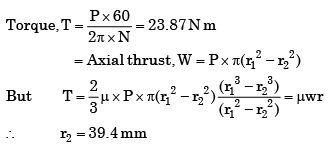QUESTION: 3

### The ratio of tension on the tight side to that on the slack side in a flat belt drive is

Solution: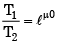QUESTION: 4

The percentage improvement in power capacity of a flat belt drive, when the wrap angle at the driving pulley is increased from 150° to 210° by an idler arrangement for a friction coefficient of 0.3, is

Solution:

We know that Power transmitted (P) = ( T1 −T2 ) .v W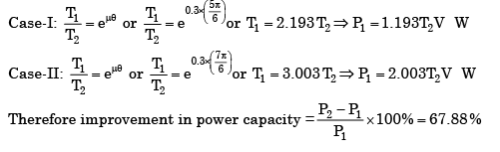QUESTION: 5

In a 6 × 20 wire rope, No.6 indicates the

Solution:

6×20 wire rope: 6 indicates number of strands in the wire rope and 20 indicates no of wire in a strand.

QUESTION: 6

Self locking in power screw is better achieved by decreasing the helix angle and increasing the coefficient of friction.

Solution:
QUESTION: 7

Which of the following stresses are associated with the design of pins in bushed pin-type flexible coupling?    1. Bearing stress

2. Bending stress

3. Axial tensile stress

4. Transverse shear stress

Select the correct answer using the codes given below

Solution:
QUESTION: 8

The bolts in a rigid flanged coupling connecting two shafts transmitting power are subjected to

Solution:

The bolts are subjected to shear and bearing stresses while transmitting torque.

QUESTION: 9

Two co-axial rotors having moments of inertia I1, I2 and angular speeds ω1 and ω2 respectively are engaged together. The loss of energy during engagement is equal to

Solution:
QUESTION: 10

Which of the following statements regarding laws governing the friction between dry surfaces are correct?    1. The friction force is dependent on the velocity of sliding.

2. The friction force is directly proportional to the normal force.

3. The friction force is dependent on the materials of the contact surfaces.

4. The frictional force is independent of the area of contact

Solution:
QUESTION: 11

When the intensity of pressure is uniform in a flat pivot bearing of radius r, the friction force is assumed to act at

Solution:
QUESTION: 12

In case of a multiple disc clutch, if n1 is the number of discs on the driving shaft and n2 is the number of discs on the driven shaft, then what is the number of pairs of contact surfaces?

Solution:
QUESTION: 13

On the motors with low starting torque, the type of the clutch to be used is

Solution:
QUESTION: 14

Match List-I with List-II and select the correct answer using the codes given below the lists: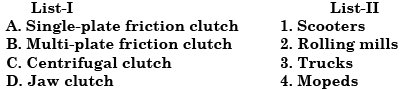Code: A B C D

Solution:
QUESTION: 15

Assertion (A): In design of arms of a pulley, in belt drive, the cross-section of the arm is, elliptical with minor axis placed along the plane of rotation.

Reason (R): Arms of a pulley in belt drive are subjected to complete reversal of stresses and is designed for bending in the plane of rotation.

Solution:
QUESTION: 16

Which one of the following belts should not be used above 40°C?

Solution:
QUESTION: 17

Assertion (A): Crowning is provided on the surface of a flat pulley to prevent slipping of the belt sideways.

Reason (R): Bell creep, which is the reason for slip of the belt sideways, is fully compensated by providing crowning on the pulley.

Solution:

Belt creep has no effect on sideways.

QUESTION: 18

Which one of the following statements relating to belt drives is correct?

Solution: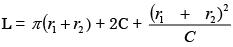where C = centre distance of shafts.

QUESTION: 19

In a Belt drive, if the pulley diameter is doubled keeping the tension and belt width constant, then it will be necessary to

Solution:

Due to twice increase in diameter of pulley, torque on key is double and has to be resisted by key width. Length can't be increased as belt width is same.

QUESTION: 20

Centrifugal tension in belts is

Solution: